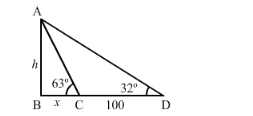# The angle of elevation of the top of a tower as observed form a point in a horizontal plane through

Question:

The angle of elevation of the top of a tower as observed form a point in a horizontal plane through the foot of the tower is 32°. When the observer moves towards the tower a distance of 100 m, he finds the angle of elevation of the top to be 63°. Find the height of the tower and the distance of the first position from the tower. [Take tan 32° = 0.6248 and tan 63° = 1.9626]

Solution:

Let h be height of tower and the angle of elevation as observed from the foot of tower is 32° and observed move towards the tower with distance 100 m then angle of elevation becomes 63°.

Let BC = x and CD = 100

Now we have to find height of tower

So we use trigonometrical ratios.In a triangle ABC,

$\Rightarrow \quad \tan C=\frac{A B}{B C}$

$\Rightarrow \quad \tan 63^{\circ}=\frac{A B}{B C}$

$\Rightarrow \quad 1.9626=\frac{h}{x}$

$\Rightarrow \quad x=\frac{h}{1.9626}$

Again in a triangle,

$\Rightarrow \quad \tan D=\frac{A B}{B C+C D}$

$\Rightarrow \quad \tan 32^{\circ}=\frac{h}{x+100}$

$\Rightarrow \quad 0.6248=\frac{h}{x+100}$

$\Rightarrow \quad x+100=\frac{h}{0.6248}$

$\Rightarrow \quad 100=\frac{h}{0.6248}-\frac{h}{1.9626}$

$\Rightarrow \quad 100=\frac{h \times 1.9626-h \times 0.6248}{0.6248 \times 1.9626}$

$\Rightarrow \quad 100=\frac{h(1.9626-0.6248)}{0.6248 \times 1.9626}$

$\Rightarrow \quad 100=\frac{h(1.3378)}{0.6248 \times 1.9626}$

$\Rightarrow \quad 100 \times 0.6248 \times 1.9626=h \times 1.3378$

$\Rightarrow \quad h=\frac{100 \times 0.6248 \times 1.9626}{1.3378}$

$\Rightarrow \quad=\frac{122.6232}{1.3378}$

$\Rightarrow \quad=91.66$

$\Rightarrow \quad x=\frac{91.66}{1.9626}$

$\Rightarrow \quad=46.7$

So distance of the first position from the tower is = 100 + 46.7 = 146.7 m

Hence the height of tower is $91.66 \mathrm{~m}$ and the desires distance is $146.7 \mathrm{~m}$.